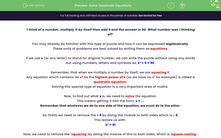### Comprehensive & curriculum aligned

In this worksheet, students will learn how to solve quadratic equations with three terms by rearranging and finding square roots as whole numbers and decimals (no factorising required).Key stage:  KS 4

Year:  GCSE

GCSE Subjects:   Maths

GCSE Boards:   Pearson Edexcel, OCR, Eduqas, AQA,

Curriculum topic:   Algebra

Curriculum subtopic:   Solving Equations and Inequalities Algebraic Equations

Difficulty level:#### Worksheet Overview

'I think of a number, multiply it by itself then add 9 and the answer is 90. What number was I thinking of?'

You may already be familiar with this type of puzzle and how it can be expressed algebraically.

These sorts of problems are best solved by writing them as equations.

If we use x (or any letter) to stand for original number, we can write the puzzle without using any words but using numbers, letters and symbols as: x² + 9 = 90

Remember, that when we multiply a number by itself, we are squaring it.

Any equation which contains an as the highest power of x (so we have no x³ for example) is called a quadratic equation.

Solving this special type of equation is a very important area of maths.

Now, to find out what x is, we need to solve the equation.

This means getting it into the form: x = ...

Remember that whatever we do to one side of the equation, we must do to the other.

So, firstly we need to remove the + 9 by doing the inverse to both sides which is - 9.

This leaves us with:

x² = 81

Now, we need to remove the 'squaring' by doing the inverse of this to both sides, which is 'square rooting'.

This gives us:

x = 9

Now strictly speaking there are two square roots of 81.

This is because when you square a negative number you get a positive, so both 9² = 81 and (-9)² = 81.

So the square root of 81 could be 9 or -9.

Usually, we are just interested in the positive square root, but it is always safest to include both.

Remember, the symbol for square root is √ so we write:

√81 = +9 or -9      or      √81 = ±9    (the ± symbol means the answer can be positive or negative)

We can set out our working like this:Let's try another example now.

Here is another equation: 2x² + 2 = 100

In order to solve this equation, first, we must subtract 2 from both sides:

2x² = 98

Now we need to be careful about the order we do the next two inverses.

2x² means 2 times , so we need to apply the inverse of × 2 next which is ÷ 2:

x² = 49

Finally, we square root both sides to give:

x = ±7

We set out our working like this:If our last step here does not have a whole number square root, we will need to use a calculator.

There are some questions like this in the activity, so you should grab a calculator before you start.

In this activity, we will solve quadratic equations with three terms by rearranging and calculating square roots of whole numbers and decimals.

### What is EdPlace?

We're your National Curriculum aligned online education content provider helping each child succeed in English, maths and science from year 1 to GCSE. With an EdPlace account you’ll be able to track and measure progress, helping each child achieve their best. We build confidence and attainment by personalising each child’s learning at a level that suits them.

Get started